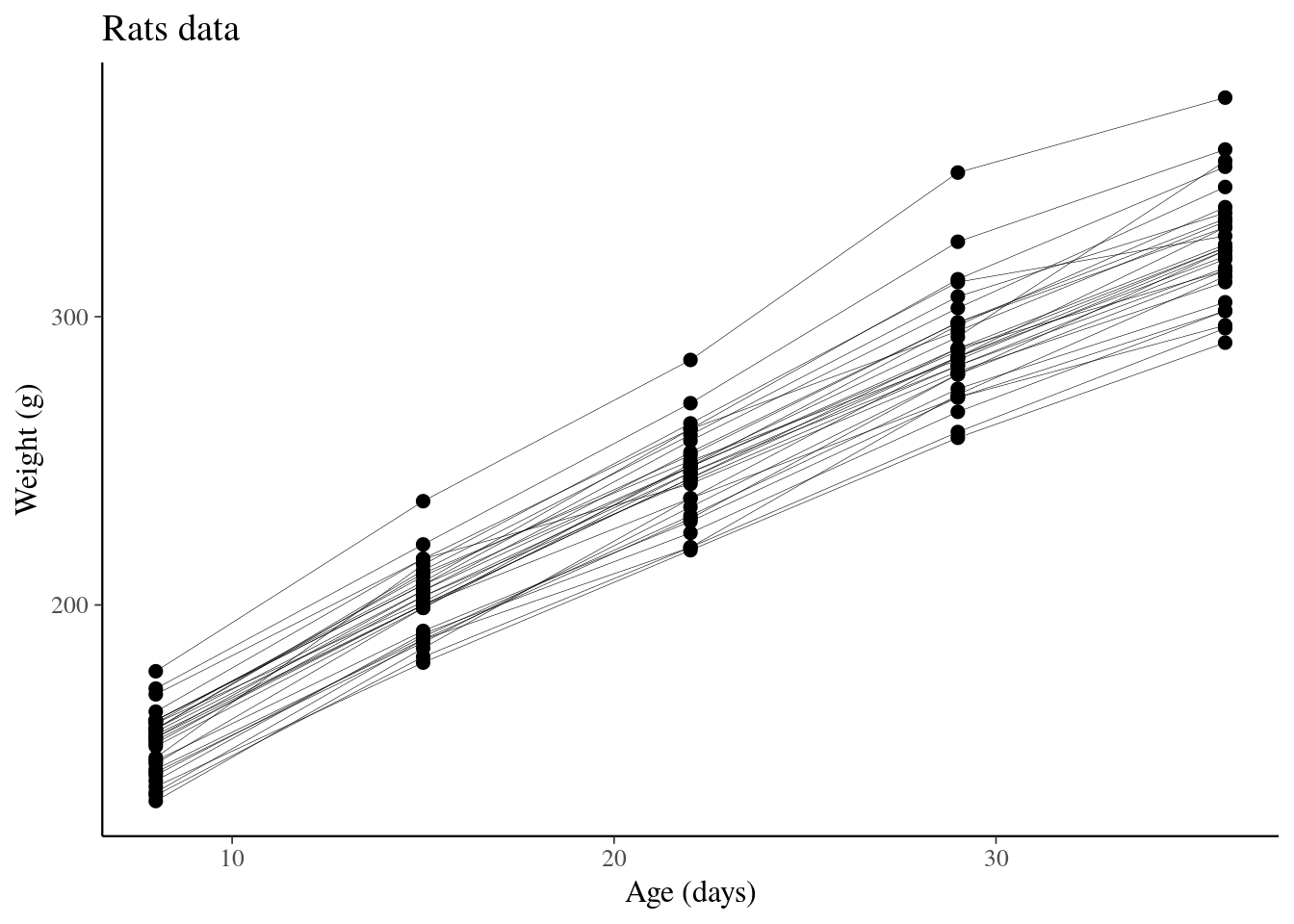# Setup

``````library("rprojroot")
root<-has_basename("modelselection")\$make_fix_file()
library("loo")
library("rstanarm")
options(mc.cores = parallel::detectCores())
library("ggplot2")
library("bayesplot")
theme_set(bayesplot::theme_default(base_family = "sans"))``````

# 1 Introduction

In this case study, we demonstrate different cross-validation variants for hierarchical/multilevel models. A related video discusses the here used examples starting at 22:53. For time series specific cross-validation, see Bürkner, Gabry and Vehtari (2019).

# 2 Data

Throughout, we will use a simple grouped data. The example data is taken from Section 6 of Gelfand et al. (1990), and concerns 30 young rats whose weights were measured weekly for five consecutive weeks.

``````sourceToList = function(file){
source(file, local = TRUE)
d = mget(ls())
d\$file = NULL
d
}
#
rats = sourceToList(root("rats.data.R"))
rats = with(rats, list(
N = N,
Npts = length(y),
rat = rep(1:nrow(y), ncol(y)),
x = rep(x, each = nrow(y)),
y = as.numeric(y),
xbar = xbar
))``````

Create dataframe

``````dfrats <- with(rats, data.frame(age=x, age_c=x-22, weight=y, rat=rat))
N <- dim(dfrats)``````

Plot data

``````pr <- ggplot(data=dfrats, aes(x=age, y=weight)) +
geom_line(aes(group=rat), color="black", size=0.1) +
geom_point(color="black", size=2) +
labs(x="Age (days)", y="Weight (g)", title="Rats data")
pr``````Just by looking at the data, it seems that if the rat growth would be modelled with a linear model (up to an age of 36 days). Individual intercepts are likely and possibly also individual slopes.

# 3 Models

We are going to compare three models: One with population effect only, another with an additional varying intercept term, and a third one with both varying intercept and slope terms.

Simple linear model

``fit_1 <- stan_glm(weight ~ age, data=dfrats, refresh=0)``

Linear model with hierarchical intercept

``fit_2 <- stan_glmer(weight ~ age + (1 | rat), data=dfrats, refresh=0)``

Linear model with hierarchical intercept and slope

``fit_3 <- stan_glmer(weight ~ age + (age | rat), data=dfrats, refresh=0)``

# 4 Leave-one-out cross-validation

In leave-one-out cross-validation (LOO), one observation is left out at a time and predicted given all the other observations.

``````pr1 <- pr +
geom_point(data=dfrats[69,], color="red", size=5, shape=1) +
ggtitle('Leave-one-out')
pr1``````